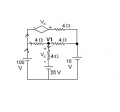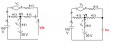Thevenin's Equivalent Circuit Concept

divinesage

Joined Sep 13, 2010
7
Hello everybody, first up I'd like to say I'm very lucky to have googled and found such nice help forum. Anyway I do have a question which I need help on, it's below:For maximum power, R(L) should be equal to R(TH) in Thevinin's Equivalent Circuit. So am I right to say that all I need to do is find R(TH) and I'll be done? I believe I have a conceptual error here as I am unable to find the answer as displayed above.

What I did was to remove all power sources from the circuit first, so then Vx would be zero since the dependent source is zero. My idea was that since Vx through the dependent source is zero, then the object that it's dependent on would also have a zero Vx. (ie. the p.d. across the 4 ohm resistor which has the Vx on it is zero and hence removed). Doing so would give me a 8/3 ohm resistance for R(TH) which is certainly not the answer.

My guess is that the dependent source plays a part in R(TH), but I was struggling over the question for a while unable to get anything resembling the 3 ohm answer above. So, any help here would be greatly appreciated. Thanks!Jony130

Joined Feb 17, 2009
5,287
Well I think that to find Rth with dependent source you need first find Vth without RL and then replaced RL by a short circuit and then you need to find this short current Isc.
And finally
Rth = Vth/Isc

•divinesage

Ghar

Joined Mar 8, 2010
655
You can't remove Vx because it does affect Rth.

The reason is that Rth is defined as V/I applied to the port you're looking at.

Jony's method works, or you can apply either a voltage (or current) at the port and find the current (or voltage). The ratio is Rth = V/I

Jony130

Joined Feb 17, 2009
5,287
or you can apply either a voltage (or current) at the port and find the current (or voltage). The ratio is Rth = V/I(100V - V1)/4Ω = (V1 - 20V)/4Ω + (V1 - 10V)/4Ω
V1 = 130/3 = 43.33V
I1 = 25/3A + 85/3A = 110/3 = 36.67A
Rth = 10V/36.67 = 0.27Ω

But if I do it "my way"Then Rth = 120V/40A = 3Ω

•divinesage

Ghar

Joined Mar 8, 2010
655
You apply the voltage or current with all independent sources set to 0, sorry.
I'll work it out later tonight.

Jony130

Joined Feb 17, 2009
5,287
You apply the voltage or current with all independent sources set to 0, sorry.
Well, now everything is correct.
Vx = 10V * 2/6 = 3.33V

I1 = 10V/6Ω + 6.67V/4Ω = 10/3A = 3.33A

Rth =10V/3.333A = 3Ω

Ghar

Joined Mar 8, 2010
655
Ok, you saved me some time, thanksdivinesage

Joined Sep 13, 2010
7

View attachment 22587

(100V - V1)/4Ω = (V1 - 20V)/4Ω + (V1 - 10V)/4Ω
V1 = 130/3 = 43.33V
I1 = 25/3A + 85/3A = 110/3 = 36.67A
Rth = 10V/36.67 = 0.27Ω

But if I do it "my way"

View attachment 22588

Then Rth = 120V/40A = 3Ω
Okay I understand both methods now. But I've been getting Rth = 60/30 = 2Ω for Jony's method. Been checking the polarities of Vx and I can't seem to find any problem with it. Very strange.Current through resistor (I'll call it I3) with Vx is negative, so the dependant voltage source Vx should be negative. If I apply KVL around the topmost loop it becomes

-Vx -8I1 + 4I2 = 0
This would lead me eventually to:
I3 = I2 (which doesn't make sense because I1 + I2 = I3 and this would make I1 = 0)

The only way which I can get the correct 120V is when Vx is a positive value but then that would mean wrong polarities in the circuit.

Thanks again for the help!

Last edited:

Jony130

Joined Feb 17, 2009
5,287
First what I did is to fond voltage on node V1.
So for current that I draw in schematics
(100V - V1)/4Ω + ( (100V+Vx) - V1)/8Ω = (V1 - 20V)/4Ω

And Vx = V1 - 20V

And for finding Isc

(100V - V1)/4Ω = (V1 - 20V)/4Ω + V1/4Ω

Jony130

Joined Feb 17, 2009
5,287
The only way which I can get the correct 120V is when Vx is a positive value but then that would mean wrong polarities in the circuit.
Why? Wrong polarities ? For me everything is ok with polarities in the circuit.

divinesage

Joined Sep 13, 2010
7
From my diagram below:

I1 + I2 = I3
Vx = 4 I2

Applying KVL around leftmost loop:

100 - 4 I1 + 4 I2 - 20 = 0
20 = I1 - I2

Applying KVL around top loop:

Vx + 4 I3 + 4 I3 + 4 I1 = 0
Vx + 12 I1 + 8 I2 = 0

Since Vx = 4 I2,

12 I1 = -12 I2
I1 = -I2
I3 = 0 (doesn't make sense)

Very strange..